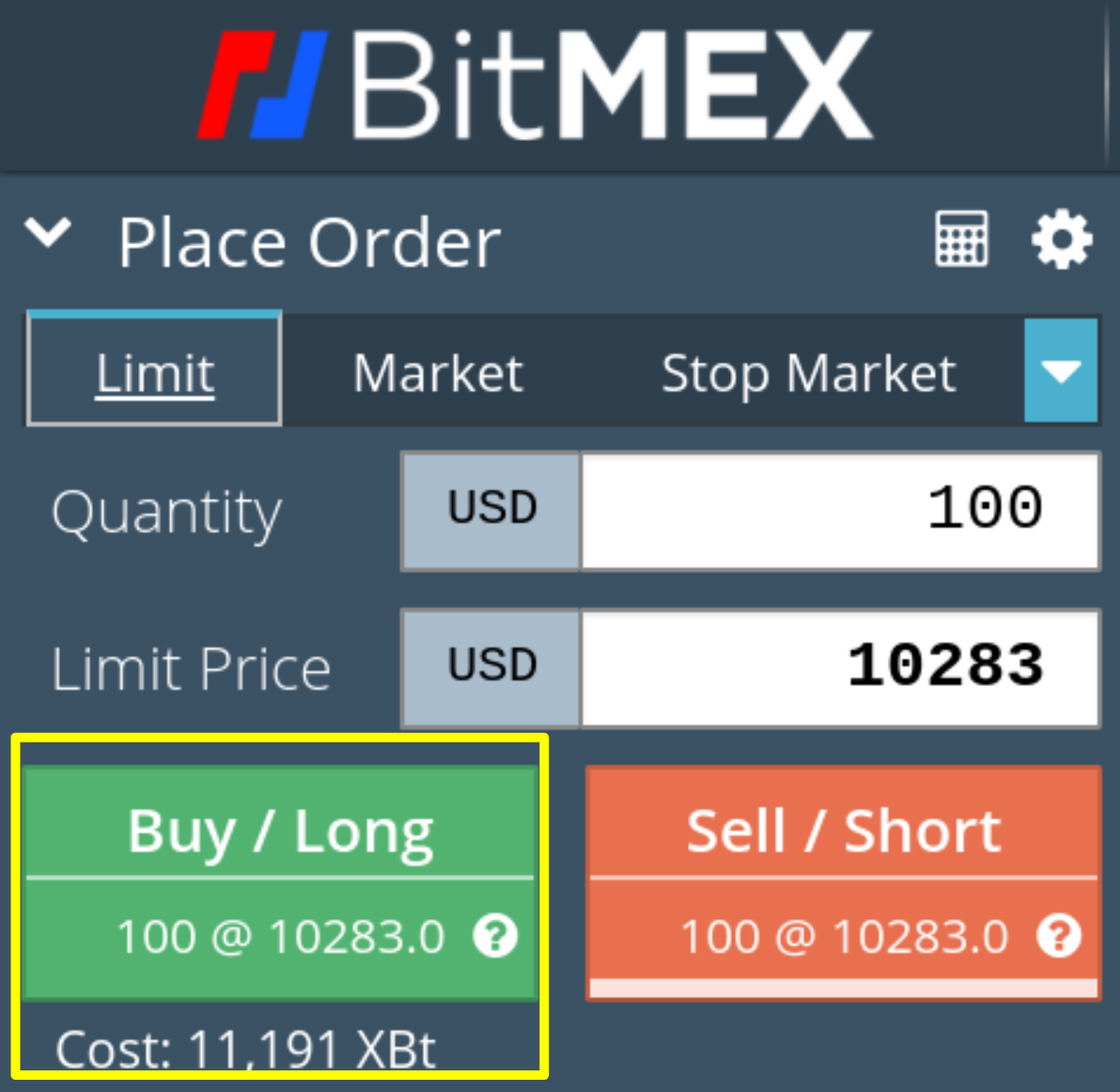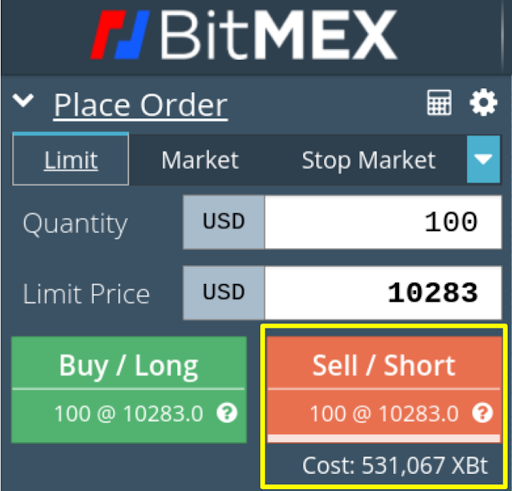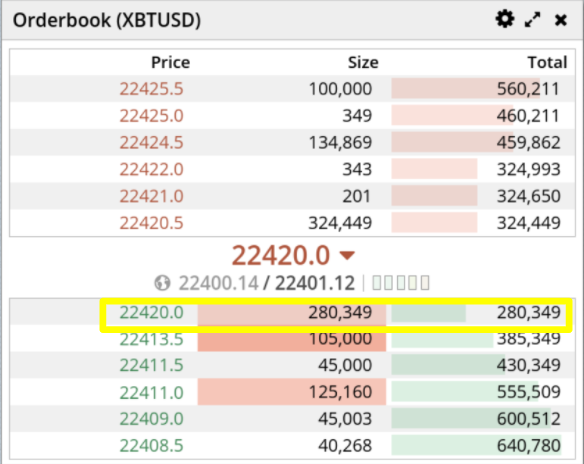Order cost is the cost required when you place your orders. The cost includes the required initial margin and trading fees.

To calculate order cost, you need to understand two key concepts.

Entry Order Value = Entry Price * Contract #

Initial Margin = 1/Leverage * Entry Order Value

The Entry Price has to be converted to the value under the relevant cryptocurrency. To find more information about how to convert the specific contract's quoted price to value under the relevant cryptocurrency, please refer to the Contracts tab on our website.

XBT value. To find more information about how to convert the specific contract’s quoted price to an XBT value, please refer to the Contracts tab on our website.(Source: https://www.bitmex.com)

In this article, we will use XBTUSD Perpetual Swap contract as an example. You can refer to the below information which you can easily find on our website as below.(Source: https://www.bitmex.com/app/seriesGuide/XBT)

To convert the price into XBT value, you can apply the calculation as shown above.

XBT Contract Value = Multiplier / XBTUSD Price = 1 / XBTUSD Price

Order Cost Calculation

1. 1/Leverage * Entry Order Value + 2 * Entry Order Value * Taker Fee

2. 1/Leverage * Entry Order Value + (Entry Order Value + Bankruptcy Value) * Taker Fee

Both 1 and 2 enables you to get the estimated order cost. 2 can get closer value to the actual order cost compared to 1.

The fee rate applied per contract varies. Please refer to the link below to check the specific contract’s fee rate that you would like to calculate the order cost manually.

https://www.bitmex.com/app/fees

Example 1 - Buy order cost calculation

For example, if you would like to buy 100 of XBTUSD perpetual swap contracts at \$10,283 with 100x leverage. The required order cost is 11,191 XBt. We can manually calculate the order cost estimates as below.1. 1/Leverage * Entry Order Value + 2 * Entry Order Value * Taker Fee

Order Cost = 1 / 100 * 0.009725 XBT + 2 * 0.009725 XBT * 0.075% = 0.00011184 XBT = 11,184 XBt

• Order Value = Entry Price * Contract # = 1/10283 * 100 = 0.00009725 * 100  = 0.009725 XBT
• Entry Order Price = Max (Ask Price, Limit Order Price)

2. 1/Leverage * Entry Order Value + (Entry Order Value + Bankruptcy Value) * Taker Fee

Order Cost = 1 / 100 * 0.009725 XBT + (0.009725 XBT * 0.075% + 0.0098225 XBT * 0.075% = 0.00011191 XBT = 11,191 XBt

• Entry Order Price = Max (Ask Price, Limit Order Price)
• Entry Order Value = Round (1/Entry Order Price, 8) * # Contract = Round (1/10283 * 100, 8) * 100 = 0.00009725 * 100 = 0.009725
• Bankruptcy Value = Entry Order Value + 1/Leverage * Entry Order Value = 0.00982225

Example 2 - Sell order cost calculation

For example, if you would like to sell 100 of XBTUSD perpetual swap contracts at \$10,283 with 100x leverage. The required order cost is 531,067 XBt. We can manually calculate the order cost estimates as below.

Mark Price at the moment is 22401.12. If the expected liquidation price of your position after the order gets executed is lower than the mark price, it means the position will be liquidated at the time of the execution due to the current mark price status. Thus, in order to have the position, Sell Premium must be added to the initial margin.3. 1/Leverage * Entry Order Value + (Entry Order Value + Bankruptcy Value) * Taker Fee rate + Sell Premium

Order Cost = 1 / 100 * 0.009725 XBT + (0.009725 XBT + 0.00982225 XBT) * 0.075% + Sell Premium = 0.00011191 XBT + 0.00519876 XBT = 0.00531067 XBT = 531,067 XBt• Entry Order Price = Max (Bid price, Limit Order Price)
• Entry Order Value = Round (1/Entry Order Price, 8) = Round(1/10283, 8) * 100 = 0.00009725 * 100 = 0.009725
• Bankruptcy Value = Entry Order Value + 1/Leverage * Entry Order Value = 0.00982225
• Mark Price Order Value = Round(1/22401.12, 8)*100 = 0.004464
• Sell Premium = Max(0,Entry Price order value - ABS(Entry Price Order Value*(1/Leverage-(Maintenance Margin rate/100-Funding rate/100)))-Mark Price Order Value) = Max(0, 0.009725 - ABS(0.009725*(0.01-(0.35/100 + 0.01/100)))-0.004464 = 0.00519876

Please note that when you use Cross Margin for your position, you need to apply the maximum leverage you can use for the specific contract. For instance, 100 is the maximum leverage available for XBTUSD Perpetual Swap contract, you use 100 for ‘Leverage’ in the calculation. 50 is the maximum leverage available for ETHUSD Perpetual Swap contract and you need to use 50 for ‘Leverage’ in the formula.